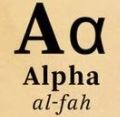Anuj Gupta
1

The tent is in the shape of a cone.

The radius of the tent is given as 7 m and the height is given as 10 m as shown below.The slant height l can be calculated from the formula l = \sqrt{r^{2}+h^{2}}

Substituting the values of r = 7 and h = 10 in the formula we get

l = \sqrt{49+100} = \sqrt{149} = 12.2 m

The surface area of the tent = \pi r l(since surface area of a cone is \pirl)

= \frac{22}{7}\times7\times 12.2 m^{2}

= 268.4m^{2}

Since the canvas covers the surface area of the tent, the area of the canvas is 268.4m^{2}

Length of the canvas = \frac{area}{width}

The width is given as 2m, and we have the area = 268.4m

So, the length of the canvas used = \frac{268.4}{2}=134.2 cm.Krishna
0

Step 1: Note down the given measurements

Radius of conical tent  (r) = 7 meters

Height  (h) = 10 meters.

Width of canvas = 2 meters

Step 2: Find the slant height of the cone

FORMULA:  Slant height l^2 = r^2 + h^2

l = \sqrt{r^2 + h^2}

l = \sqrt{7^2 + 10^2}

l = \sqrt{49 + 100}

l = \sqrt{149} = 12.2 m

Step 3: Calculate the surface area of the conical tent

FORMULA:  Surface area of the conical tent = \pi rl

= \frac{22}{7} * 7 * 12.2 m^2

= 268.4 m^2

Step 4: Determine the length of canvas used in making the tent.

Area of the tent = 268.4 m^2

Length * width = 268.4 m^2

Length = \frac{268.4}{width}

Length = \frac{268.4}{2}

Length = 134.2 m